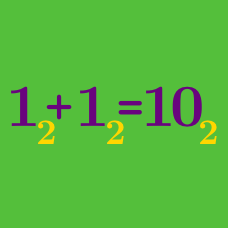Number Theory

# Number Bases: Level 2 Challenges

$\Large \color{#20A900}{200_5} \quad \color{#3D99F6}{100_7} \quad \color{#69047E}{56_9}$ $\text{Which of these numbers is the largest?}$

Note: $200_5$ means that the number is in base $5$, and so forth.$\large{\begin{array}{ccccc} &1& 1& 0 & 0&1\\ + & &1 & 0 & 1&1\\ \hline \end{array}}$

Compute the binary sum above. Be sure to give your answer in binary.

A new prime has been discovered: $\Large {2}^{74,207,281} - 1.$ Find the sum of its digit when written in Binary (base 2).

What is the greatest 4 digit perfect square in base 7? (Input your answer in base 7).

What number follows 666 in base 7?

×